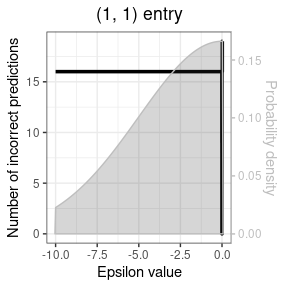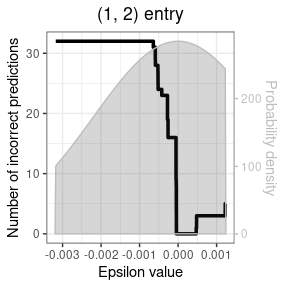# Quick start

• Install PressPurt
# Specify branch and build vignettes
devtools::install_github("dkoslicki/PressPurt",
subdir = "R_version",
build_vignettes = FALSE)
• Check available python versions
• Set python with previously made conda environment
• Install python dependencies
• Run PreprocessMatrix
• Run ComputeEntryWisePerturbationExpectation
• Plot
• specific figures
• all figures
library(PressPurt)
find_python()
#> Default Python:
#>  /home/gibbond/anaconda3/envs/r-reticulate/bin/python
#>
#>  Python versions found:
#>  /home/gibbond/anaconda3/envs/r-reticulate/bin/python /usr/bin/python /usr/bin/python3 /home/gibbond/anaconda3/bin/python /home/gibbond/.virtualenvs/bleh/bin/python /home/gibbond/.virtualenvs/PressPurt/bin/python /home/gibbond/.virtualenvs/Test/bin/python /home/gibbond/anaconda3/envs/bleh/bin/python /home/gibbond/anaconda3/envs/last-test/bin/python /home/gibbond/anaconda3/envs/lpi-asms/bin/python /home/gibbond/anaconda3/envs/pb-assembly/bin/python /home/gibbond/anaconda3/envs/picrust2/bin/python /home/gibbond/anaconda3/envs/PressPurtEnv/bin/python /home/gibbond/anaconda3/envs/r-tensorflow/bin/python /home/gibbond/anaconda3/envs/test_r_versions/bin/python /home/gibbond/anaconda3/envs/test-env/bin/python
#>
#>  List of condaenvironments:
#>  : ,
#>
#>  List of virtualenvs:
#>  bleh PressPurt Test
set_python_virtual("PressPurt", verbose = TRUE)
#>
#>  Setting virtual environment
#>
#>  Python/virtual environment in use:
#> python:         /home/gibbond/.virtualenvs/PressPurt/bin/python
#> libpython:      /home/gibbond/anaconda3/envs/r-reticulate/lib/libpython3.6m.so
#> pythonhome:     /home/gibbond/anaconda3/envs/r-reticulate:/home/gibbond/anaconda3/envs/r-reticulate
#> version:        3.6.7 |Anaconda, Inc.| (default, Oct 23 2018, 19:16:44)  [GCC 7.3.0]
#> numpy:          /home/gibbond/.virtualenvs/PressPurt/lib/python3.6/site-packages/numpy
#> numpy_version:  1.16.3
#>
#> NOTE: Python version was forced by use_python function
py_depend(virtualenv = "PressPurt")
# Path to your matrix - in this case a test dataset that comes with the package
infile <- system.file("extdata", "Modules", "IGP.csv", package = "PressPurt")
MultiPert <- ComputeMultiEntryPerturbationExpectation(input_file = infile)
PreProsMatrix <- PreprocessMatrix(input_file = infile,
output_folder = NULL,
max_bound = 10,
Entrywise <- ComputeEntryWisePerturbationExpectation(PreProsMatrix = PreProsMatrix,
distribution_type="truncnorm",
list_of_numswitch_to_plot <- list(c(1, 1), c(1, 2))
GenerateEntryWiseFigures(EntryWise=Entrywise,
all_numswitch_plots = FALSE,
list_of_numswitch_to_plot=list_of_numswitch_to_plot)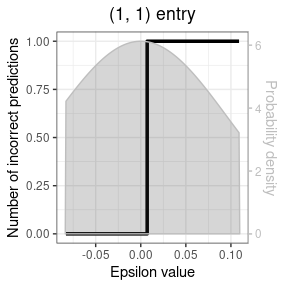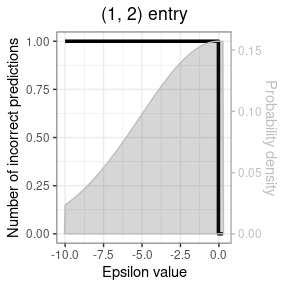GenerateEntryWiseFigures(EntryWise=Entrywise,
all_numswitch_plots = TRUE)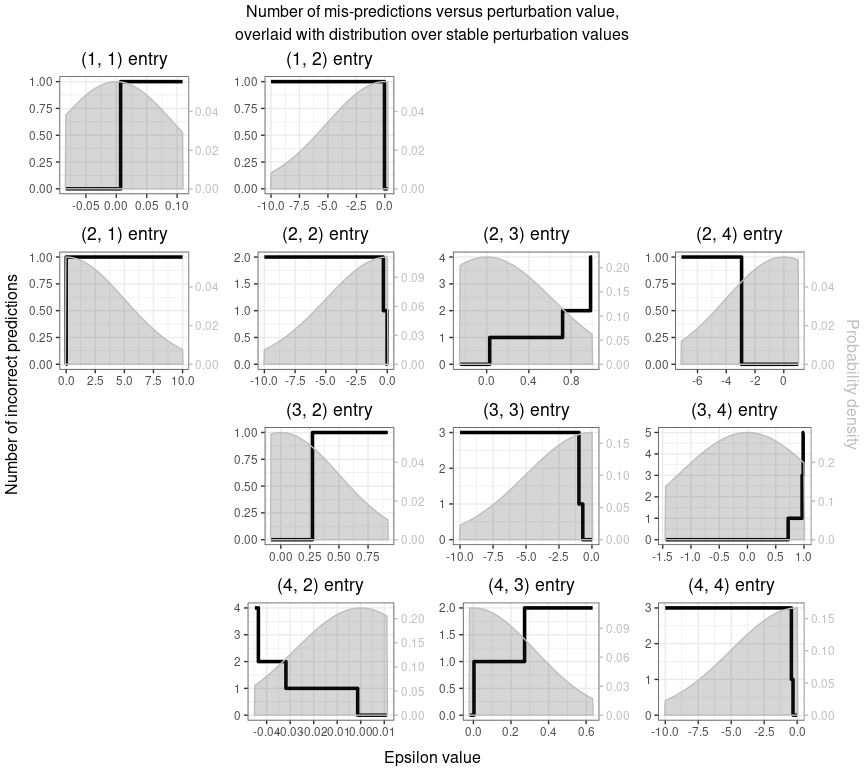This package uses python through the R package reticulate. You’ll need to install the needed python libraries into a conda env or a virtualenv. The “dependencies_tutorial” walks you through how to do this. Once this is done, you’ll need to load your conda env or virtualenv.

Note: If you want to change conda envs or virtual envs, you’ll need to restart R because of how reticulate works.

# set the conda env
set_python_virtual("PressPurtEnv", verbose = TRUE)

# Example: IGP.csv

When running this workflow, one may keep everything in R memory or save the output and intermediate files to a specified folder. However, many of these output files are python objects. Let’s start by looking at the R-based version:

## R-object based

### Compute Multi Entry Perturbation Expectation

This function takes a jacobian matrix and computes the multi-entry perturbation expectation.

In this example we take the IGP.csv matrix and use the default num_iterates and interval_length values.

The IGP.csv file is an example dataset that is included in the PressPurt package.

infile <- system.file("extdata", "Modules", "IGP.csv", package = "PressPurt")
MultiPert <- ComputeMultiEntryPerturbationExpectation(input_file = infile)
MultiPert
#>  0.266

### PreprocessMatrix

This function pre-processes a matrix by figuring out what the intervals of asymptotic stability are, as well as finding which perturbation values lead to a sign switch.

In this example we use a max_bound of 10, don’t perturb zero entries and use 2 threads.

PreProsMatrix <- PreprocessMatrix(input_file = infile,
max_bound = 10,
zero_perturb = FALSE,
threads = 2)

#### Output

The output is a list of 9 objects which includes: original_matrix, matrix_size, column_names, row_names, non_zero, num_switch_functions_py, asymptotic_stability_start, asymptotic_stability_end, num_switch_funcs_r

##### Matrix

This includes the original matrix and information about that matrix:

# Original Matrix
PreProsMatrix$original_matrix #> [,1] [,2] [,3] [,4] #> [1,] -0.237 -1.000 0.000 0.000 #> [2,] 0.100 -0.015 -1.000 -1.000 #> [3,] 0.000 0.100 -0.015 -1.000 #> [4,] 0.000 0.045 0.100 -0.015 # Matrix size PreProsMatrix$matrix_size
#>  4
# row names
PreProsMatrix$row_names #>  "0" "1" "2" "3" # column names PreProsMatrix$column_names
#>  "0" "1" "2" "3"
# number of entries that aren't zero
PreProsMatrix$non_zero #>  12 ##### Asymptotic of Stability intervals The asymptotic of stability intervals are divided up into two matrices: start and stop. # Start PreProsMatrix$asymptotic_stability_start
#>             [,1]         [,2]         [,3]       [,4]
#> [1,] -0.08298658  -9.99999999   0.00000000   0.000000
#> [2,] -0.02593439  -9.99999999  -0.25212423  -7.117953
#> [3,]  0.00000000  -0.08404049  -9.99999999  -1.448616
#> [4,]  0.00000000  -0.04499999  -0.02042571 -10.000000
# End
PreProsMatrix$asymptotic_stability_end #> [,1] [,2] [,3] [,4] #> [1,] 0.1090182 0.25934395 0.00000000 0.00000000 #> [2,] 10.0000000 0.01499999 0.99999999 0.99999999 #> [3,] 0.0000000 0.91947448 0.01499999 0.99999999 #> [4,] 0.0000000 0.01113441 0.63448010 0.01499999 # To access entry 1,1: PreProsMatrix$asymptotic_stability_start[1,1]
#>  -0.08298658
PreProsMatrix$asymptotic_stability_end[1,1] #>  0.1090182 ##### Num Switch The num_switch_funcs object is a list of each non-zero entry in the matrix and the corresponding perturbation values that leads to a sign switch. For example, entry 1,1 has 1 sign switch and 2,2 has 2. # get the length of the list, see it's # the same as the non-zero entries length(PreProsMatrix$num_switch_funcs_r)
#>  12
# see the names of the entries
names(PreProsMatrix$num_switch_funcs_r) #>  "(1, 1)" "(1, 2)" "(2, 1)" "(2, 2)" "(2, 3)" "(2, 4)" "(3, 2)" "(3, 3)" #>  "(3, 4)" "(4, 2)" "(4, 3)" "(4, 4)" # num switch function for 1,1 PreProsMatrix$num_switch_funcs_r$(1, 1) #> num_switch_val start end #> 1 1 0.007114943 0.1090182 # num switch function for 2,2 PreProsMatrix$num_switch_funcs_r$(2, 2) #> num_switch_val start end #> 1 1 -0.3125131 -0.01305907 #> 2 2 -10.0000000 -0.31251310 You can ignore the num_switch_functions_py object as it is a python dictionary that is passed to the next step. ### Compute Entry Wise Perturbation Expectation This function computes the expected number of sign switches from perturbing each entry individually. In this example, the PreProsMatrix object is passed, “truncnorm” distribution is used, input_a is 0 and input_b is -2. Entrywise <- ComputeEntryWisePerturbationExpectation(PreProsMatrix = PreProsMatrix, distribution_type="truncnorm", input_a=0, input_b=-2, threads=1) #### Output The output includes the same object that were found in PreProsMatrix with 3 additions: original_matrix, matrix_size, column_names, row_names, non_zero, num_switch_functions_py, asymptotic_stability_start, asymptotic_stability_end, num_switch_funcs_r, expected_num_switch, distributions_object, ns_object_plot ##### Expected num switch This is a data frame of expected sign switches. Entrywise$expected_num_switch
#>            0          1          2           3
#> 0 0.03155098 0.05964785 0.00000000 0.000000000
#> 1 0.06219858 0.12130032 0.05215649 0.021873797
#> 2 0.00000000 0.03050608 0.15946639 0.008706269
#> 3 0.00000000 0.05350997 0.08104533 0.174734087
##### Distributions Object

This object gets the probability density distribution into a plot ready format for each non-zero interval in the matrix. By default there are 250 points in the distribution.

# distributions object of each non-zero entry in the matrix
names(Entrywise$distributions_object) #>  "(1, 1)" "(1, 2)" "(2, 1)" "(2, 2)" "(2, 3)" "(2, 4)" "(3, 2)" "(3, 3)" #>  "(3, 4)" "(4, 2)" "(4, 3)" "(4, 4)" # position in the matrix Entrywise$distributions_object$(1, 1) #>$position
#>  1 1
# Interval of asymptotic of stability
Entrywise$distributions_object$(1, 1)
#> $interval #>  -0.08298658 0.10901820 # ready to plot probability density distribution dataframe head(Entrywise$distributions_object$(1, 1)[], n = 10) #> x_range dist_vals #> 1 -0.08375460 0.000000 #> 2 -0.08297733 4.217092 #> 3 -0.08220006 4.246567 #> 4 -0.08142278 4.275968 #> 5 -0.08064551 4.305290 #> 6 -0.07986824 4.334529 #> 7 -0.07909097 4.363681 #> 8 -0.07831369 4.392740 #> 9 -0.07753642 4.421704 #> 10 -0.07675915 4.450566 ##### Num Switch Object The ns_object_plot is the Num Switch functions that have been converted into step functions and are ready to plot! # Step function for each non-zero entry in the matrix names(Entrywise$ns_object_plot)
#>   "(1, 1)" "(1, 2)" "(2, 1)" "(2, 2)" "(2, 3)" "(2, 4)" "(3, 2)" "(3, 3)"
#>   "(3, 4)" "(4, 2)" "(4, 3)" "(4, 4)"
# entry 1,1
Entrywise$ns_object_plot$(1, 1)
#>            nsx nsy
#> 1 -0.082986581   0
#> 2  0.007114943   0
#> 3  0.109018199   1
# entry 4,2
Entrywise$ns_object_plot$(4, 2)
#>            nsx nsy
#> 6 -0.044999990   4
#> 5 -0.043500000   4
#> 4 -0.043500000   3
#> 3 -0.031798604   2
#> 2 -0.001305907   1
#> 1  0.011134411   0

The y-0 position has been added to the step function:

# Before
Entrywise$num_switch_funcs_r$(1, 1)
#>   num_switch_val       start       end
#> 1              1 0.007114943 0.1090182
# After - step function
Entrywise$ns_object_plot$(1, 1)
#>            nsx nsy
#> 1 -0.082986581   0
#> 2  0.007114943   0
#> 3  0.109018199   1

### Generate Entry Wise Figures

The GenerateEntryWiseFigures function plots the Num Switch step function on the Y-axis in black and the probability density on the Y-axis in grey.

One may plot individual entries or a list of entries with the list_of_numswitch_to_plot option:

list_of_numswitch_to_plot <- list(c(1, 1), c(1, 2))
GenerateEntryWiseFigures(EntryWise=Entrywise,
all_numswitch_plots = FALSE,
list_of_numswitch_to_plot=list_of_numswitch_to_plot)Or plot all figures with all_numswitch_plots.

For large matrices it is not recommended that you plot them all in one plot.

GenerateEntryWiseFigures(EntryWise=Entrywise,
all_numswitch_plots = TRUE)## Save to disk

### Basic pipeline

One may instead save objects to disk if one sets an output_folder. The following files will be created in the specified folder:

• asymptotic_stability.npy
• distributions.pkl
• num_non_zero.npy
• row_names.txt
• column_names.txt
• expected_num_switch.csv
• num_switch_funcs.pkl
• size.npy

If you are going to be doing multiple runs in the same folder it is recommended that you specify a file prefix - this will add _ to the beginning of every file.

# Not run in vignette creation
PreProsMatrix <- PreprocessMatrix(input_file = infile,
output_folder = "test_r/test4",
prefix = "IGP", max_bound = 10, threads = 2)
Entrywise <- ComputeEntryWisePerturbationExpectation(input_folder = "test_r/test4",
prefix = "IGP",
distribution_type="truncnorm",

list_of_numswitch_to_plot <- list(c(1, 1), c(1, 2))
GenerateEntryWiseFigures(input_folder = "test_r/test4",
prefix = "IGP",
all_numswitch_plots = FALSE,
list_of_numswitch_to_plot=list_of_numswitch_to_plot)

Many of them are numpy or pickle python objects. But the GenerateEntryWiseFigures can handle them!

### Process Data

One may also turn the data saved to a folder into an R friendly format with process_data. This function will put the data in the same format as the output as ComputeEntryWisePerturbationExpectation.

Entrywise_from_disk <- process_data(matrix = infile,
type = "csv",
folder = "test_r/test4",
prefix = "IGP")
# Path to the E.coli matrix
infile <- system.file("extdata", "Real", "Ecoli.csv", package = "PressPurt")
PreProsMatrix <- PreprocessMatrix(input_file = infile,
output_folder = NULL,
max_bound = 10,
list_of_numswitch_to_plot=list_of_numswitch_to_plot)